# 小白学数据：教你用Python实现简单监督学习算法

• f表示学生学习时间和考试成绩之间的关系
• X表示输入（学习小时数）
• Y表示输出（考试分数）
• C表示随机误差

• K近邻
• 决策树
• 朴素贝叶斯
• 支持向量机

pip install pandas
pip install matplotlib
pip install scikit-learn

from sklearn import datasets
import pandas as pd
import matplotlib.pyplot as plt

# Prints the type/type object of iris
print(type(iris))
# <class 'sklearn.datasets.base.Bunch'>

# prints the dictionary keys of iris data
print(iris.keys())

# prints the type/type object of given attributes
print(type(iris.data), type(iris.target))

# prints the no of rows and columns in the dataset
print(iris.data.shape)

# prints the target set of the data
print(iris.target_names)

X = iris.data

Y = iris.target

# Convert datasets' type into dataframe
df = pd.DataFrame(X, columns=iris.feature_names)

# Print the first five tuples of dataframe.
print(df.head())

<class ‘sklearn.datasets.base.Bunch’>
dict_keys([‘data’, ‘target’, ‘target_names’, ‘DESCR’, ‘feature_names’])]
<class ‘numpy.ndarray’> <class ‘numpy.ndarray’>
(150, 4)
[‘setosa’ ‘versicolor’ ‘virginica’]
sepal length (cm) sepal width (cm) petal length (cm) petal width  (cm)
0   5.1   3.5   1.4  0.2
1   4.9   3.0   1.4  0.2
2   4.7   3.2   1.3  0.2
3   4.6   3.1   1.5  0.2
4   5.0   3.6   1.4  0.2

Sklearn中的K最近邻算法

K近邻分类器就是一个懒惰学习法。

K近邻基于类比学习，比较一个测试样本和与之相似训练集数据。训练集有n个属性表征。每个样本由n维空间中的一个点表示。这样，训练集中的所有样本都储存在n维模式空间中。当给定一个未知的样本，K近邻分类器在模式空间中搜寻和未知样本最接近的k个训练样本。这k个训练样本就是未知样本的k个近邻。

“接近度”用距离来度量，例如欧几里得距离。较好的K值可以通过实验确定。

from sklearn import datasets
from sklearn.neighbors import KNeighborsClassifier

# Load iris dataset from sklearn

# Declare an of the KNN classifier class with the value with neighbors.
knn = KNeighborsClassifier(n_neighbors=6)

# Fit the model with training data and target values
knn.fit(iris['data'], iris['target'])

# Provide data whose class labels are to be predicted
X = [
[5.9, 1.0, 5.1, 1.8],
[3.4, 2.0, 1.1, 4.8],
]

# Prints the data provided
print(X)

# Store predicted class labels of X
prediction = knn.predict(X)

# Prints the predicted class labels of X
print(prediction)

[1 1]

KNN对IRIS数据集分类的直观可视化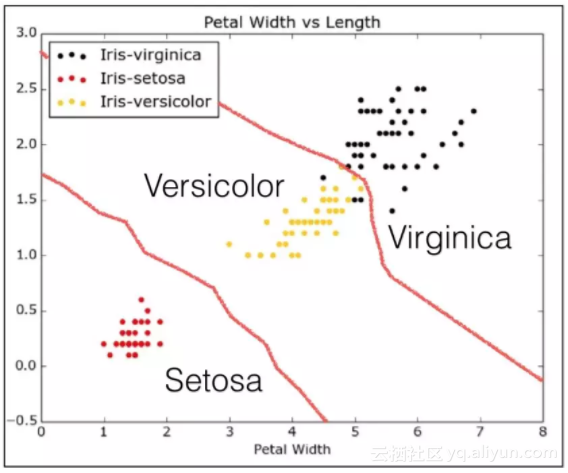• 线性回归
• 逻辑回归
• 多项式回归

βo是截距
β1是斜率
e是误差项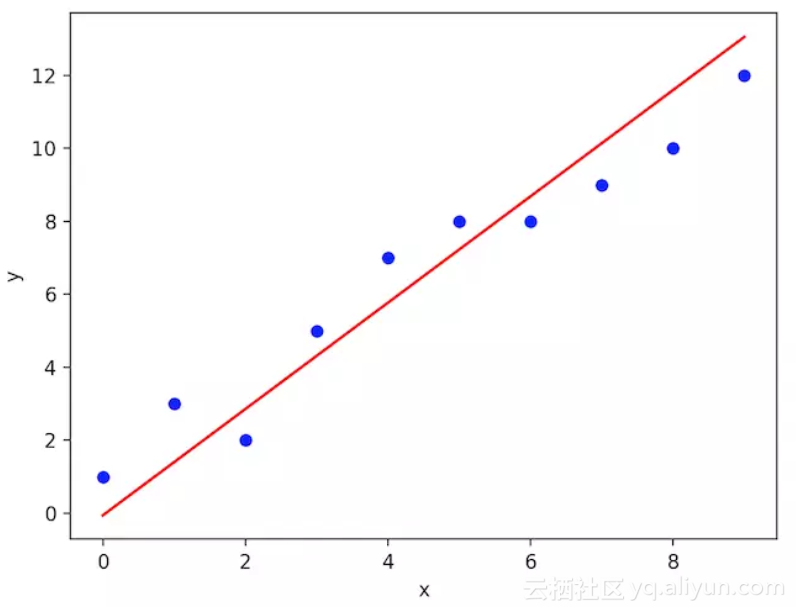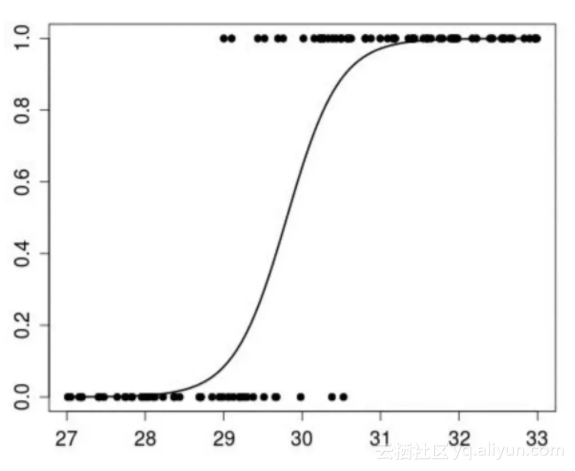from sklearn import datasets, linear_model

import matplotlib.pyplot as plt import numpy as np # Load the diabetes dataset diabetes = datasets.load_diabetes() # Use only one feature for training diabetes_X = diabetes.data[:, np.newaxis, 2] # Split the data into training/testing sets diabetes_X_train = diabetes_X[:-20] diabetes_X_test = diabetes_X[-20:] # Split the targets into training/testing sets diabetes_y_train = diabetes.target[:-20] diabetes_y_test = diabetes.target[-20:] # Create linear regression object regr = linear_model.LinearRegression() # Train the model using the training sets regr.fit(diabetes_X_train, diabetes_y_train) # Input data print('Input Values') print(diabetes_X_test) # Make predictions using the testing set diabetes_y_pred = regr.predict(diabetes_X_test) # Predicted Data print("Predicted Output Values") print(diabetes_y_pred) # Plot outputs plt.scatter(diabetes_X_test, diabetes_y_test, color='black') plt.plot(diabetes_X_test, diabetes_y_pred, color='red', linewidth=1) plt.show()

[
[ 0.07786339]  [-0.03961813]  [ 0.01103904]  [-0.04069594]
[-0.03422907]  [ 0.00564998]  [ 0.08864151]  [-0.03315126]
[-0.05686312]  [-0.03099563]  [ 0.05522933]  [-0.06009656]
[ 0.00133873]  [-0.02345095]  [-0.07410811]  [ 0.01966154]
[-0.01590626]  [-0.01590626]  [ 0.03906215]  [-0.0730303 ]
]

[
225.9732401   115.74763374  163.27610621  114.73638965
120.80385422  158.21988574  236.08568105  121.81509832
99.56772822   123.83758651  204.73711411   96.53399594
154.17490936  130.91629517   83.3878227   171.36605897
137.99500384  137.99500384  189.56845268   84.3990668
]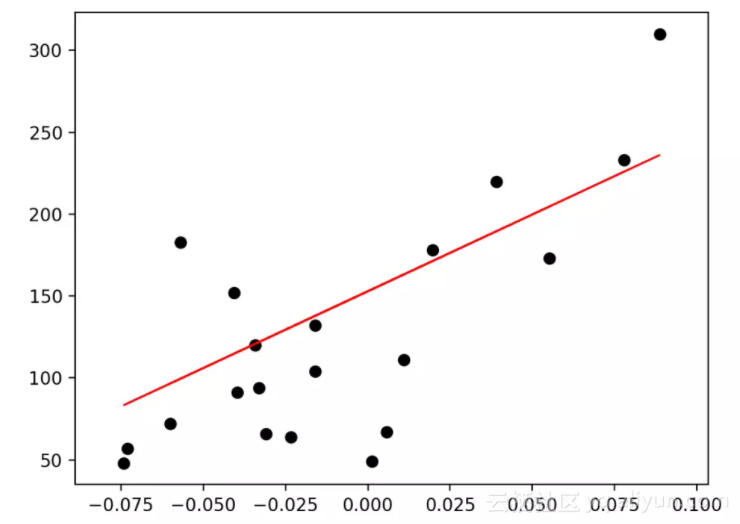• Scikit-Learn
• Tensorflow
• Pytorch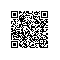使用钉钉扫一扫加入圈子
+ 订阅